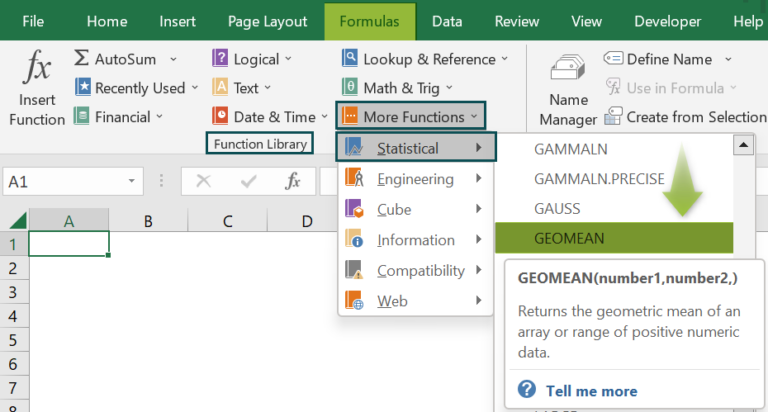# GEOMEAN Excel FunctionArticle byExcelMojo Team## What Is GEOMEAN Function In Excel?

The Geometric Mean or the GEOMEAN Excel function calculates the average growth rate or the rate of return with variable rates in a given dataset. The geometric mean is different from the arithmetic mean and is always less than the arithmetic mean/average.

The GEOMEAN function in Excel is an inbuilt “Statistical” function, so we can insert the formula from the “Function Library” or enter it directly in the worksheet. For example, we will calculate the GEOMEAN function for the values in the image below.

Select cell B2, enter the formula =GEOMEAN(A2:A3), and press “Enter”.

The result is ‘14.142’, as shown below.

###### Key Takeaways
• The GEOMEAN Excel function calculates the geometric average/mean of an array or cell range of positive numbers that are greater than zero.
• The arguments can be numbers, names, arrays, or cell references that contain numbers.
• If there are empty cells and cells that contain text or logical values, the function ignores them and calculates the rest.
• The function can take up to 255 different arguments, such as numbers, cell references, ranges, arrays, and constants.
• The mathematical formula for geometric mean is shown below, i.e., for a set of numbers [y1, y2, …, yn], the geometric mean is the nth root of the product of the n numbers

### GEOMEAN() Excel Formula

The syntax of the GEOMEAN Excel formula is,

The argument of the GEOMEAN Excel formula is,

• number1: It is a mandatory argument. These are the cell range with numeric values to calculate the geometric mean.
• [number2],…: These are optional arguments. These are the series of cell ranges with numeric values to calculate the geometric mean.

### How To Use GEOMEAN Excel Function?

We can use the GEOMEAN function in 2 ways, namely,

1. Access from the Excel ribbon.
2. Enter in the worksheet manually.

#### Method #1 – Access from the Excel ribbon

Choose an empty cell for the output 🡪 select the “Formulas” tab 🡪 go to the “Function Library” group 🡪 click the “More Functions” option drop-down 🡪 click the “Statistical” option right arrow 🡪 select the “GEOMEAN” function, as shown below.

The “Function Arguments” window appears. Enter the arguments in the “Number1”, and “Number2” fields 🡪 click “OK”, as shown below.

#### Method #2 – Enter in the worksheet manually

1. Select an empty cell for the output.
2. Type =GEOMEAN( in the selected cell. [Alternatively, type =G or =GE and double-click the GEOMEAN function from the list of suggestions shown by Excel.]
3. Enter the arguments as cell values or Excel cell references and close the brackets.
4. Press the “Enter” key.

Let us take a basic example to understand its application.

We will calculate the geometric average using GEOMEAN Excel function.

In the table, the data is,

• Column A, B, & C contains the Data.
• Column D contains the Output.

The steps to calculate using GEOMEAN Excel function are,

1. Select cell D2, and enter the formula =GEOMEAN(A2:A5, i.e., the numeric value.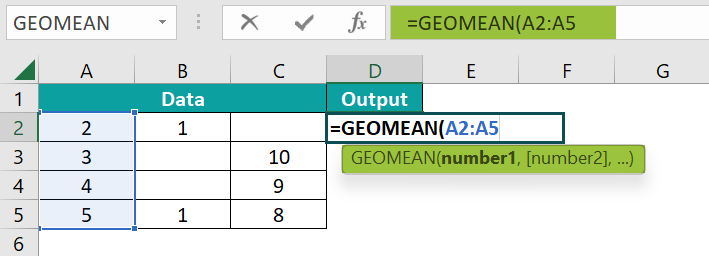2. Enter the value of ‘number2’ as B2:B5. The formula now is =GEOMEAN(A2:A5,B2:B5,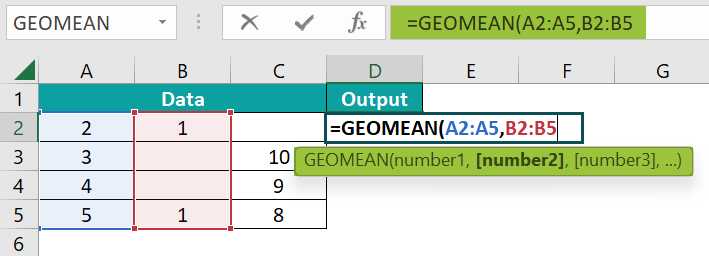3. Enter the value of ‘number3’ as C2:C5, i.e., the numeric value, and close the brackets. The complete formula is =GEOMEAN(A2:A5,B2:B5,C2:C5)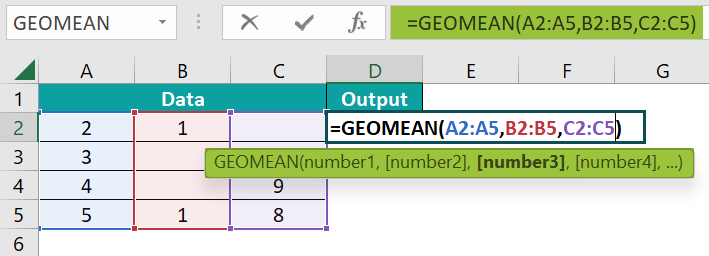4. Press the “Enter” key. The result is “3.5359”, as shown below.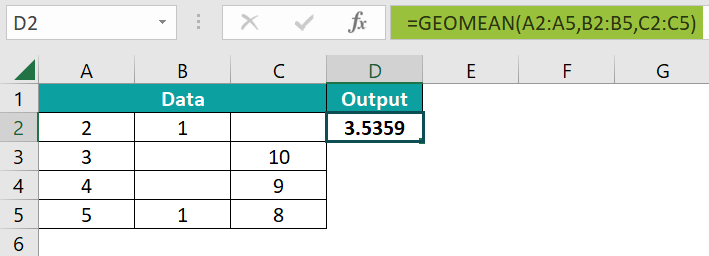### Examples

We will understand some advanced scenarios using the GEOMEAN Excel function examples.

#### Example #1

We will calculate the average using the GEOMEAN function for the data in percentage.

In the table, the data is,

• Column A contains the Rates.
• Column B displays the calculated Output.

The procedure to calculate using the GEOMEAN formula is,

Select cell B2, enter the formula =GEOMEAN(A2:A6), and press the “Enter” key.

The result is “24%”, as shown above.

#### Example #2

We will calculate the average using the GEOMEAN function for the data in decimal.

In the table, the data is,

• Column A contains the Numbers.
• Column B displays the calculated Output.

The procedure to calculate using the GEOMEAN formula is,

Select cell B2, enter the formula =GEOMEAN(A2:A5), and press the “Enter” key.

The result is “2.4347”, as shown above.

#### Example #3

We will calculate the error using the GEOMEAN function for the data.

In the table, the data is,

• Column A contains the Data.
• Column B displays the calculated Output.

The procedure to calculate using the GEOMEAN formula is,

Enter the formula =GEOMEAN(A2:A3) in cell B2, =GEOMEAN(“abc”) in cell B3, and press the “Enter” key after entering each formula

The results are a “#NUM!” error in cell B2, and a “#VALUE!” error in cell B3, as shown above. Column C is for our reference.

### Important Things To Note

• The “#NUM!” error occurs when,
• The provided values are less than or equal to zero.
• One or more cell values are negative numbers.
• The “#VALUE!” error occurs when,
• The supplied value is non-numeric.
• One or more cell values are an error or contain an error value.

1. What does the GEOMEAN Excel function mean?

The GEOMEAN Excel function returns the average rate of return of a set of values calculated using the product of the terms.

The syntax of the GEOMEAN function is =GEOMEAN(number1,[number2],…)

For example, we will calculate the average using the GEOMEAN function for the negative values.

In the table, the data is,
Column A contains the Value.
Column B displays the calculated Output.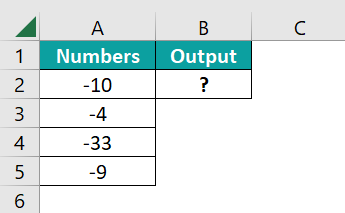The procedure to calculate the value by the GEOMEAN formula is,
Select cell B2, enter the formula =GEOMEAN(A2:A5), and press the “Enter” key.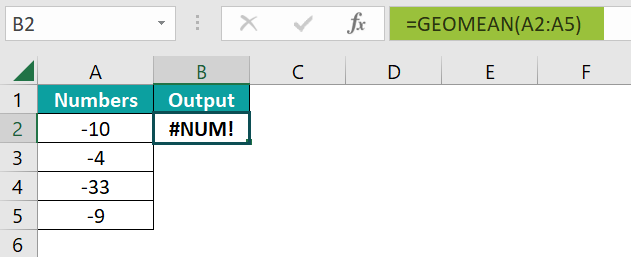The result is the “#NUM!” error that occurs when a negative value is entered, as shown above.

2. How to insert the GEOMEAN Excel function?

We can insert the GEOMEAN function as follows:
1. Select an empty cell for the output.
2. Type =GEOMEAN( in the selected cell. [Alternatively, type =G or =GE and double-click the GEOMEAN function from the list of suggestions shown by Excel.]
3. Enter the arguments as cell values or cell references and close the brackets.
Press the “Enter” key.

For example, we will calculate the average using the GEOMEAN function for the values.
In the table, the data is,
Column A contains the Value.
Column B displays the calculated Output.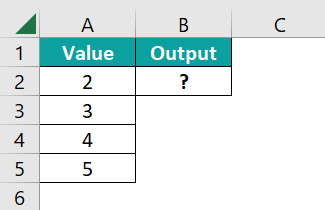The procedure to calculate the value by the GEOMEAN formula is,
Select cell B2, enter the formula =GEOMEAN(A2:A5), and press the “Enter” key.
The result is “3.3098”, as shown below.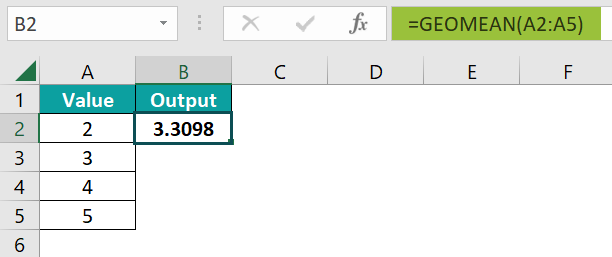3. Where is the GEOMEAN Excel function?

The GEOMEAN Excel function is found in the “Formulas” tab, as shown below.

Choose an empty cell for the output 🡪 select the “Formulas” tab 🡪 go to the “Function Library” group 🡪 click the “More Functions” option drop-down 🡪 click the “Statistical” option right arrow 🡪 select the “GEOMEAN” function, as shown below.